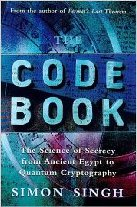Caesar Cipher

Introduction §

The Caesar cipher is one of the earliest known and simplest ciphers. It is a type of substitution cipher in which each letter in the plaintext is 'shifted' a certain number of places down the alphabet. For example, with a shift of 1, A would be replaced by B, B would become C, and so on. The method is named after Julius Caesar, who apparently used it to communicate with his generals.

More complex encryption schemes such as the Vigenère cipher employ the Caesar cipher as one element of the encryption process. The widely known ROT13 'encryption' is simply a Caesar cipher with an offset of 13. The Caesar cipher offers essentially no communication security, and it will be shown that it can be easily broken even by hand.

Example §

To pass an encrypted message from one person to another, it is first necessary that both parties have the 'key' for the cipher, so that the sender may encrypt it and the receiver may decrypt it. For the caesar cipher, the key is the number of characters to shift the cipher alphabet.

Here is a quick example of the encryption and decryption steps involved with the caesar cipher. The text we will encrypt is 'defend the east wall of the castle', with a shift (key) of 1.

plaintext:  defend the east wall of the castle
ciphertext: efgfoe uif fbtu xbmm pg uif dbtumf

It is easy to see how each character in the plaintext is shifted up the alphabet. Decryption is just as easy, by using an offset of -1.

plain:  abcdefghijklmnopqrstuvwxyz
cipher: bcdefghijklmnopqrstuvwxyza

Obviously, if a different key is used, the cipher alphabet will be shifted a different amount.

Mathematical Description §

First we translate all of our characters to numbers, 'a'=0, 'b'=1, 'c'=2, ... , 'z'=25. We can now represent the caesar cipher encryption function, e(x), where x is the character we are encrypting, as:

Where k is the key (the shift) applied to each letter. After applying this function the result is a number which must then be translated back into a letter. The decryption function is :

Plaintext

shift:

Ciphertext

Other Implementations §

For Caesar cipher code in various programming languages, see the Implementations page.

To encipher your own messages in python, you can use the pycipher module. To install it, use pip install pycipher. To encipher messages with the Caesar cipher (or another cipher, see here for documentation):

>>>from pycipher import Caesar
>>>Caesar(key=1).encipher('defend the east wall of the castle')
'EFGFOEUIFFBTUXBMMPGUIFDBTUMF'
>>>Caesar(key=1).decipher('EFGFOEUIFFBTUXBMMPGUIFDBTUMF')
'DEFENDTHEEASTWALLOFTHECASTLE'

Cryptanalysis §

See Cryptanalysis of the Caesar Cipher for a way of automatically breaking this cipher.

Cryptanalysis is the art of breaking codes and ciphers. The Caesar cipher is probably the easiest of all ciphers to break. Since the shift has to be a number between 1 and 25, (0 or 26 would result in an unchanged plaintext) we can simply try each possibility and see which one results in a piece of readable text. If you happen to know what a piece of the ciphertext is, or you can guess a piece, then this will allow you to immediately find the key.

If this is not possible, a more systematic approach is to calculate the frequency distribution of the letters in the cipher text. This consists of counting how many times each letter appears. Natural English text has a very distinct distribution that can be used help crack codes. This distribution is as follows:

This means that the letter e is the most common, and appears almost 13% of the time, whereas z appears far less than 1 percent of time. Application of the Caesar cipher does not change these letter frequencies, it merely shifts them along a bit (for a shift of 1, the most frequent ciphertext letter becomes f). A cryptanalyst just has to find the shift that causes the ciphertext frequencies to match up closely with the natural English frequencies, then decrypt the text using that shift. This method can be used to easily break Caesar ciphers by hand.

If you are still having trouble, try the cryptanalysis section of the substitution cipher page. All strategies that work with the substitution cipher will also work with the Caesar cipher (but methods that work on the Caesar cipher do not necessarily work on the general substitution cipher).

For a method that works well on computers, we need a way of figuring out which of the 25 possible decryptions looks the most like English text. See Cryptanalysis of the Caesar Cipher for a walkthrough of how to break it using quadgram statistics. The key (or shift) that results in a decryption with the highest likelyhood of being English text is most probably the correct key. Of course, the more ciphertext you have, the more likely this is to be true (this is the case for all statistical measures, including the frequency approach above). So the method used is to take the ciphertext, try decrypting it with each key, then see which decryption looks the best. This simplistic method of cryptanalysis only works on very simple ciphers such as the Caesar cipher and the rail fence cipher, even slightly more complex ciphers can have far too many keys to check all of them.

ContentsThe Code Book: The Science of Secrecy from Ancient Egypt to Quantum Cryptography ASIN/ISBN: 978-1857028799 Simon Singh's 'The Code Book' is an excellent introduction to ciphers and codes Buy from Amazon.com